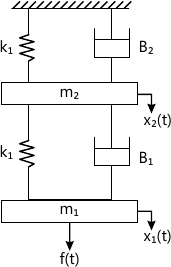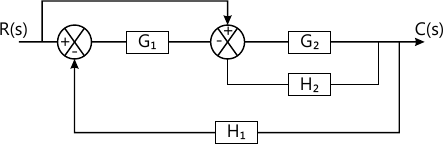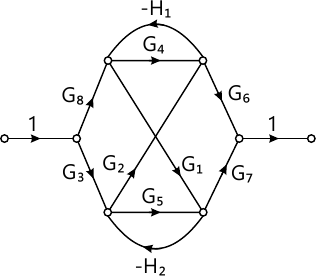Total marks: --
Total time: --
INSTRUCTIONS
(1) Assume appropriate data and state your reasons
(2) Marks are given to the right of every question
(3) Draw neat diagrams wherever necessary

1 (a) Distinguish between open loop and closed loop control system, with suitable examples.
4 M
1 (b) What are the ideal requirements of control system?
6 M
1 (c) What is Control Action? Briefly explain proportional, proportional plus derivative and proportional plus derivative plus integral controllers, with the help of block diagrams.
10 M

2 (a) Obtain the differential equation for the mechanical system shown in fig. Q2(a) and draw the equivalent mechanical system, also draw the analogous electrical network based on i) Force - voltage analogy ii) Force - current analogy.10 M
2 (b) Derive the transfer function of an armature controlled DC motor. The field current is maintained constant during operation. Assume that the armature coil has back emf $e_b = k_b \dfrac {d\theta} {dt}$ and the coil current produces a torque T=KmI on the rotor, Kb and Km are the back emf constant and motor torque constant respectively.
10 M

3 (a) Reduce the block diagram shown in fig Q3(a) to its simplest possible form and find its closed loop transfer function.10 M
3 (b) Using Mason's gain formula, find the gain of the following system shown in fig. Q3(b).10 M

4 (a) Derive an expression for the unit step response of first order system.
8 M
4 (b) A unity feedback system is characterized by an open loop transfer function $$G(s) = \dfrac {K} {s(s+10)}$$ . Determine the gain K, so that the system will have a damping ratio of 0.5. For this value of k determine peak time, setting time and peak overshoot for a unit step input.
8 M
4 (c) Ascertain the stability of the system given by the characteristics equation S5+4S4+12S3+20S2+30S+100=0, using R-H criteria.
4 M

5 (a) Sketch the polar plot for the transfer function. $G(s) = \dfrac {10} {s(s+1)(s+2)} .$
10 M
5 (b) Apply Nyquist stability criterion to the system wit transfer function. $G(s) H(s) = \dfrac {4s+1} {s^2 (1+s)(1+2s) }$ and ascertain its stability.
10 M

6 Sketch the Bode plot for $G(s) H(s) = \dfrac {2} {s(s+1)(1+0.2s) }.$ Also obtain gain margin and phase margin and crossover frequencies.
20 M

7 Sketch the root locus plot for $G(s) H(s) = \dfrac {K} {s(s+2)(s+4)(s+6) } .$ For what values of K the system becomes unstable?
20 M

8 (a) Explain the following: i) Lead compensator ii) Lag compensaor.
12 M
8 (b) Determine the state controllability and observability of the system described by $\dot x= \begin{bmatrix} -3 &1 &1 \\-1 &0 &1 \\0 &0 &1 \end{bmatrix}x+ \begin{bmatrix} 0 &1 \\0 &0 \\2 &1 \end{bmatrix} u \\ Y=\begin{bmatrix} 0 &0 &1 \\1 &1 &0 \end{bmatrix}x .$
8 M

More question papers from Control Engg